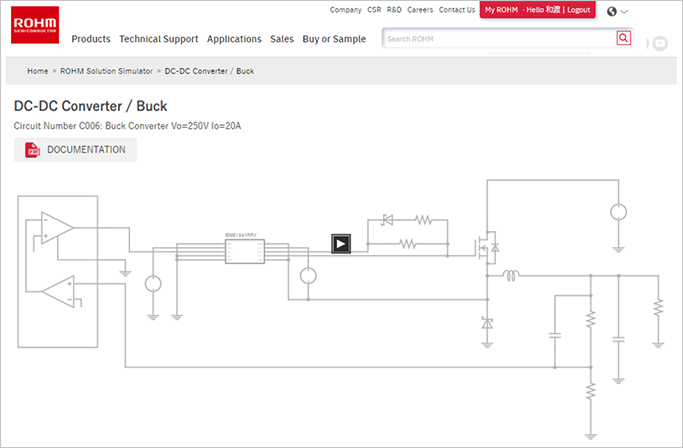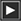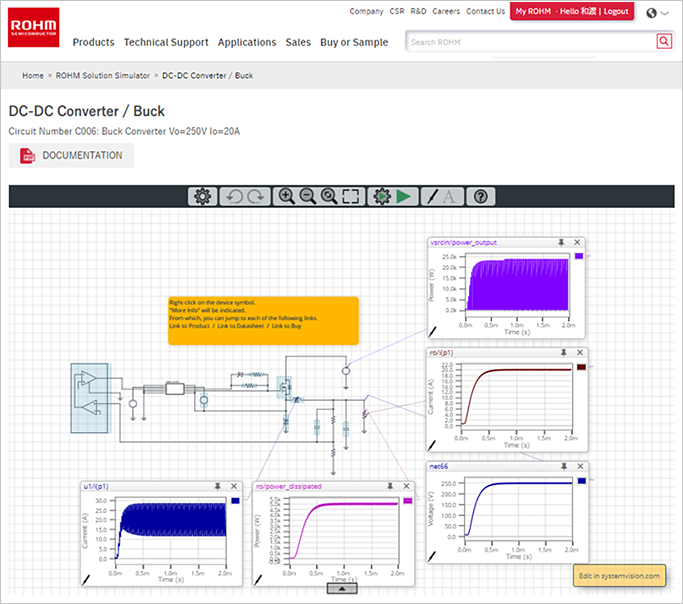Technical Information Site of Power Supply Design

2021.12.08 Simulation

# Starting a Simulation Circuit in the ROHM Solution Simulator

In the previous articles, we explained how to use the ROHM Solution Simulator in order to give the reader some understanding of what it is, and so we omitted many details. From this article, we will explain basic operations, settings, methods for displaying results, and other issues. Those persons who want to acquire the basics of operation and the like quickly can instead consult the "Hands-On User’s Manual（PDF）".

## Starting a Simulation Circuit in the ROHM Solution Simulator

We will open an actual Solution Circuit to explain operation and other matters, and so we begin by starting the simulation circuit. As an example, we will use "C6: Buck Converter Vo=250 V Io=20 A" among the Power Device Solution Circuits for DC-DC converters. The method of accessing the Solution Circuit has already been explained (see "How to Access the ROHM Solution Simulator").

Go to the ROHM Solution Simulator web page, open DC-DC Converters in the Power Device Solution Circuit category, and click on the "Simulation" button for C6. If you are not already logged in to MyROHM, you will be asked to log in. After clicking the "Simulation" button, the following circuit diagram is displayed (Fig. 1). *To directly execute the C6 simulation, click this link →
https://www.rohm.com/solution-simulator/buck_converter_vo250v_io20a

Fig. 1. Screen appearing when the C6 simulation circuit "Simulation" button is clickedUpon clicking the Run symbol () near the center of the circuit diagram, the ROHM Solution Simulator starts, and the screen switches to a display of graphs of simulation results and other information for the default simulation circuit (Fig. 2).

Fig. 2. ROHM Solution Simulator startup and simulation screen for the selected circuitWith this, the simulation circuit has been started. Next, we will explain the user interface.

#### Key Points:

・Upon clicking the "Simulation" button for a Solution Circuit of the ROHM Solution Simulator, the circuit is displayed.

・Click the Run symbol () near the center of the circuit diagram to start the ROHM Solution Simulator. Simulation results for the default simulation circuit are displayed.